Courses

# EDC And Analog

## 25 Questions MCQ Test Mock Tests Electronics & Communication Engineering GATE 2020 | EDC And Analog

Description
This mock test of EDC And Analog for GATE helps you for every GATE entrance exam. This contains 25 Multiple Choice Questions for GATE EDC And Analog (mcq) to study with solutions a complete question bank. The solved questions answers in this EDC And Analog quiz give you a good mix of easy questions and tough questions. GATE students definitely take this EDC And Analog exercise for a better result in the exam. You can find other EDC And Analog extra questions, long questions & short questions for GATE on EduRev as well by searching above.
QUESTION: 1

Solution:
QUESTION: 2

Solution:
QUESTION: 3

### Consider an n-type semiconductor in which the dopant concentration ND is 1014/cm3. If the intrinsic concentration of semiconductor 1010/cm3 then the concentration of electrons (n) and holes (P) at equillibrium are

Solution:
QUESTION: 4

Consider the following statements regarding fermi level in an extrinsic semiconductor.

1. Fermi level moves towards the centre of energy band gap as we increase the temperature.

2. In n-type semiconductor the fermi level moves towards the vallence band as we increases the doping concentration.

3. In p-type semiconductor the fermi level moves towards the conduction band as we increases the doping concentration.

Which of the following is/are correct?

Solution:
QUESTION: 5

The junction capacitance of an abrupt pn junction is 10 pf at a reverse bias voltage of 3 volt. Determine the value of capacitance when a reverse bias voltage of 15 volt is applied, if the built in potential of junction is 1 volt.

Solution:
QUESTION: 6

In the circuit shown, diodes D1, D2 and D3 are ideal and the inputs E1, E2 and E3 are 0 V for logic ‘0’ and 5V for logic ‘1’. What logic gate does the circuit represent?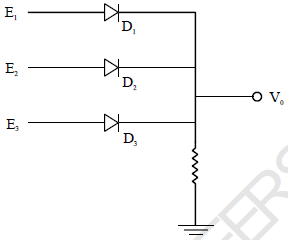Solution:
QUESTION: 7

In the given circuit, the moving-coil meter gives full-scale deflection reading when the average current
flowing through it is 1mA. The moving coil meter has internal resistance of 50?. Find the value of R that results meter a full scale deflection.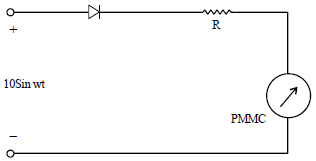Solution:
QUESTION: 8

Assume both the diodes and both the capacitors are ideal. The value of V0 in steady state is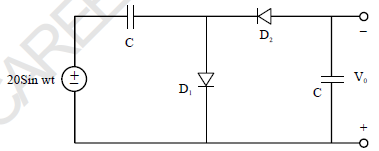Solution:
QUESTION: 9

Assume the diode is ideal, determine the current ‘i’ in the given circuit.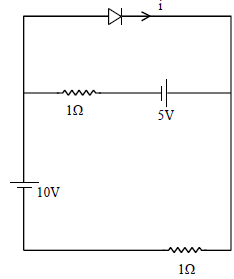Solution:
QUESTION: 10

Consider the following statements.

1. The PIV in a HWR with capacitive filter becomes 2 Vm

2. Ripple voltage is decreases as we increases the time period of input ac signal.

3. Conduction time of diode is decreases as we increase the value of capacitor.

Which of the following is/are correct?

Solution:
QUESTION: 11

In the given circuit both the transistors are perfectly matched the value fo I2 is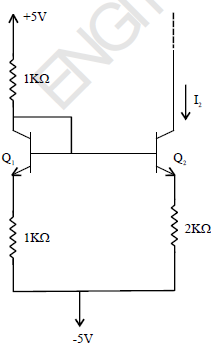Solution:
QUESTION: 12

The forward threshold voltage of each diode in the given circuit is 1 Volt and reverse breakdown voltage of each diode is 10 Volt. Determine ‘i’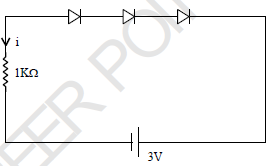Solution:
QUESTION: 13

In the given circuit the VCE (sat) for each transistor is 0. The inputs A, B and C are 0 for logic 0 and they
are 5V for logic 1. The logic gate realized by the circuit. Assume β = 100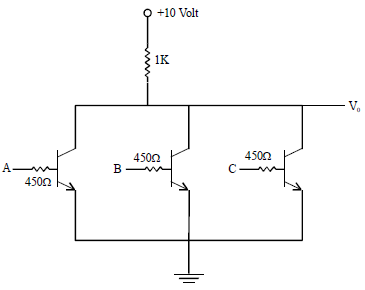Solution:
QUESTION: 14

Assume β of the transistor is 100, determine the value of V0 in the given circuit.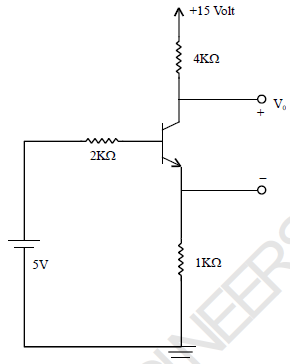Solution:
QUESTION: 15

In the ac circuit shown {The DC biasing circuit is omitted), the two BJTs are biased in active region and
have identical parameters with β >> 1. The open circuit small signal voltage gain is approximately.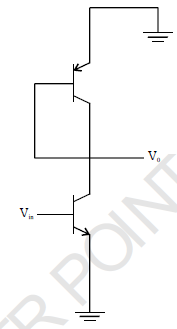Solution:
QUESTION: 16

In the circuit shown below, assume both the diodes and opamp are ideal. Determine the frequency of the oscillation of the signal obtained at V0.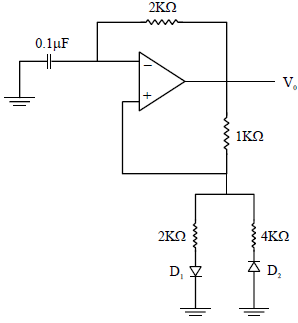Solution:
QUESTION: 17

In the given circuit, assume opamp is ideal. Determine the type and 3-dB frequency of the filter.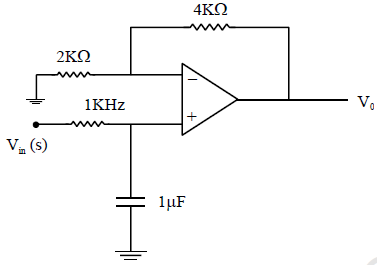Solution:
QUESTION: 18

For the CMOS circuit shown below the parameters for the MOSFET are given below:
For NMOS : Vth = 0.7V,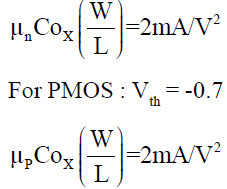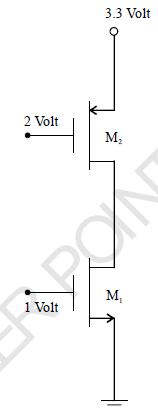Determine region of MOSFET M1 and M2.

Solution:
QUESTION: 19

For the MOSFET shown in the circuit below has Vth = 1 Volt,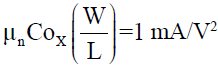. Determine the resistance offered by the MOSFET.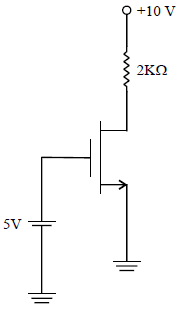Solution:
QUESTION: 20

Which of the following statement is not correct about a power amplifier?

Solution:
QUESTION: 21

Determine the duty cycle of the square wave generated at the output of terminal 3: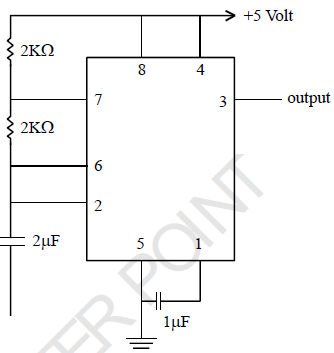Solution:
QUESTION: 22

A light of wavelength 4000A is incident on a semiconductor material having energy band gap is 1.4eV and electron hole pairs are generated. Determine the velocity of electron that is generated due to this illumination.

Solution:
QUESTION: 23

In the given circuit the opamp is ideal.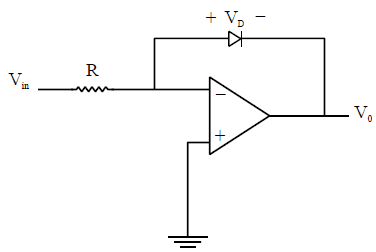This circuit is popularly known as,

Solution:
QUESTION: 24

Adding an emitter resistor to a common-emitter amplifier causes:

Solution:
QUESTION: 25

A non-inverting op-amp is shown below {assume ideal op-amp}. For
Vin = (2 + Sin 100t) Volt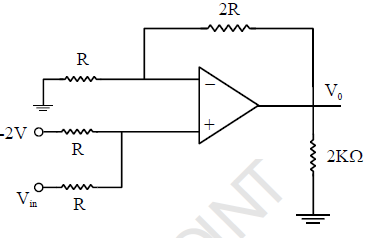The output voltage V0 for an input

Solution: